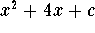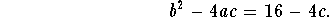#### Exercise 3.

For which values of c does the polynomialhave two complex conjugate roots?

The polynomial will have two complex conjugate roots if and only if its discriminant is negative. Since a=1 and b=4, the polynomial's discriminant is given byThe discriminant is negative, when c>4.

The polynomial will have two complex conjugate roots for c>4.[Back] [Exercises] [Next]
[Algebra] [Trigonometry] [Complex Variables]
[Calculus] [Differential Equations] [Matrix Algebra]S.O.S MATHematics home page

Do you need more help? Please post your question on our S.O.S. Mathematics CyberBoard.Helmut Knaust
Tue Jun 24 09:48:17 MDT 1997